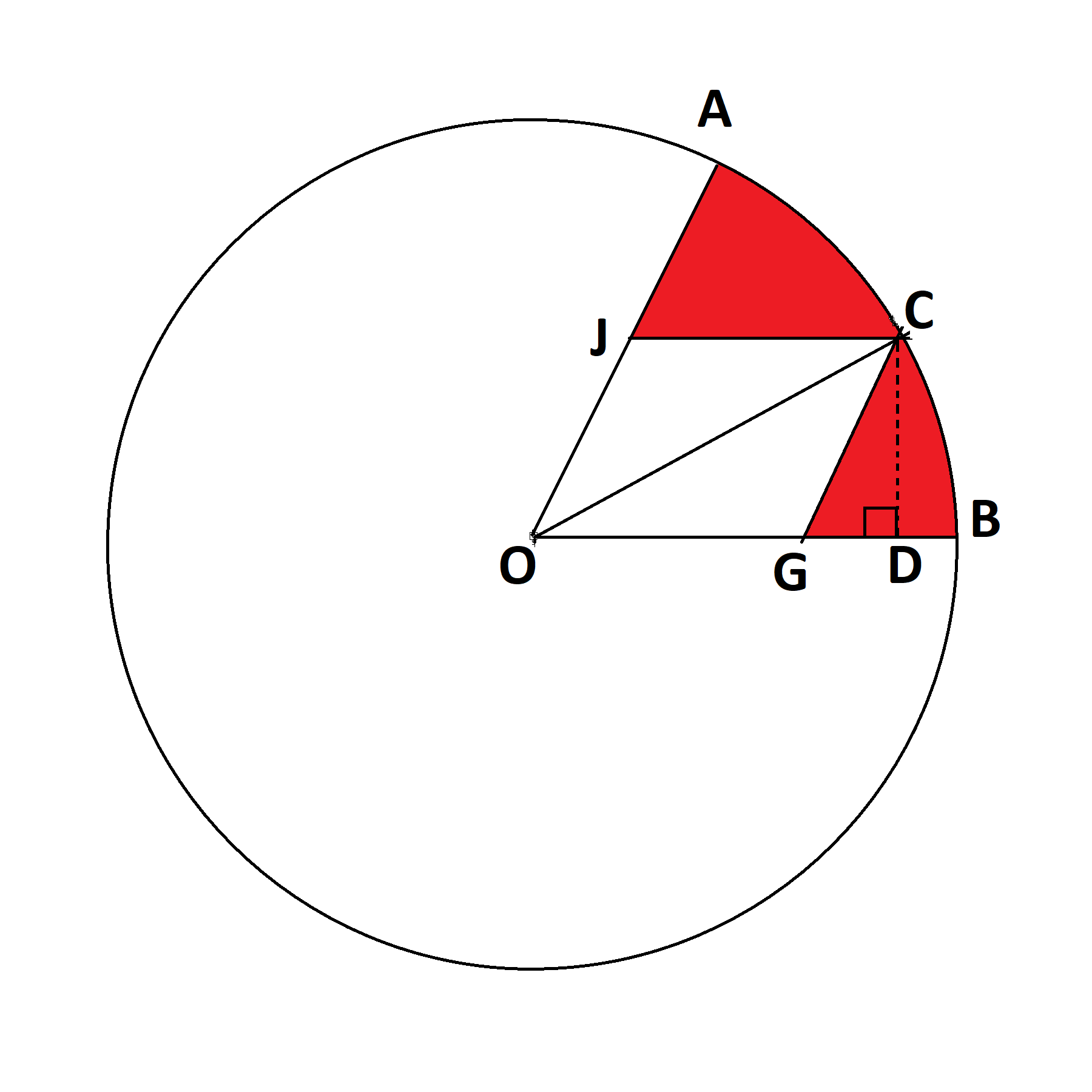# On Area percentage -1

In the Discussion I will generalise a case one of which is in this question .In $\parallel_{gm} JOGC$ assuming $\overline{JC}=\overline{CG}$ the $\red{red}$ area is $\frac{100}{\pi}(\angle COG - \frac{\sin^2(\angle COG)}{\sin(2\angle COG)})\%$ of the whole circle(all the angles are measured in radians only)

Proof:

Let us denote $\angle COG=\alpha$ overall the proof (to make things easier to write for me)

And let $\overline{JC}=\overline{CG}=a;\overline{OC}=r$

Now as $JOCG$ is a parallelogram $\therefore$ $\overline{JO} || \overline{CG} \Rightarrow \angle JOG=\angle CGD$

Also as the diagonal of a parallelogram bisects its vertex angles ${2\alpha=\angle CGD}$

In $\triangle CGD$ : $\sin(\angle CGD)=\sin(2\alpha)=\frac{\overline{CD}}{a}$ $\Rightarrow \overline{CD}=a\sin(2\alpha)$ In $\triangle COD$ : $\sin(\alpha)=\frac{\overline{CD}}{r}$ $\overline{CD}=r\sin(\alpha)$ $\Rightarrow r\sin(\alpha)=a\sin(2\alpha)$ $\Rightarrow r=a\frac{\sin(2\alpha)}{\sin(\alpha)}$ Area of $||_{gm}JOGC=A_1=\overline{OG}\times\overline{CD}=a\times a\sin(2\alpha)=a^2\sin(2\alpha)$

Area of circle $=A_2=\pi r^2=\pi a^2\frac{\sin^2(2\alpha)}{\sin^2(\alpha)}$

Area of the sector $\frac{\angle JOG}{2\pi}A=\frac{\cancel{2}\alpha}{\cancel{2}\pi}A=\frac{\alpha}{\pi}A=\frac{\alpha}{\cancel{\pi}}(\cancel{\pi} a^2\frac{\sin^2(2\alpha)}{\sin^2(\alpha)})=a^2\alpha \frac{\sin^2(2\alpha)}{\sin^2(\alpha)}$

Area of the $\red{red}$ region $=A-A_1$

Percentage of the area to the circles area$=\frac{A-A_1}{A_2}\times 100=(a^2\alpha \frac{\sin^2(2\alpha)}{\sin^2(\alpha)}-a^2\sin(2\alpha))\times \frac{100\sin^2(\alpha)}{\pi a^2\sin^2(2\alpha)}=\boxed{\frac{100}{\pi}({\alpha}-\frac{\sin^2(\alpha)}{\sin(2\alpha)})}$

Bonus:I have proved it for the case when all sides of the parallelogram are equal, you can find a formula for general case quadrilateralNote by Zakir Husain
1 year, 1 month ago

This discussion board is a place to discuss our Daily Challenges and the math and science related to those challenges. Explanations are more than just a solution — they should explain the steps and thinking strategies that you used to obtain the solution. Comments should further the discussion of math and science.

When posting on Brilliant:

• Use the emojis to react to an explanation, whether you're congratulating a job well done , or just really confused .
• Ask specific questions about the challenge or the steps in somebody's explanation. Well-posed questions can add a lot to the discussion, but posting "I don't understand!" doesn't help anyone.
• Try to contribute something new to the discussion, whether it is an extension, generalization or other idea related to the challenge.

MarkdownAppears as
*italics* or _italics_ italics
**bold** or __bold__ bold
- bulleted- list
• bulleted
• list
1. numbered2. list
1. numbered
2. list
Note: you must add a full line of space before and after lists for them to show up correctly
paragraph 1paragraph 2

paragraph 1

paragraph 2

[example link](https://brilliant.org)example link
> This is a quote
This is a quote
    # I indented these lines
# 4 spaces, and now they show
# up as a code block.

print "hello world"
# I indented these lines
# 4 spaces, and now they show
# up as a code block.

print "hello world"
MathAppears as
Remember to wrap math in $$ ... $$ or $ ... $ to ensure proper formatting.
2 \times 3 $2 \times 3$
2^{34} $2^{34}$
a_{i-1} $a_{i-1}$
\frac{2}{3} $\frac{2}{3}$
\sqrt{2} $\sqrt{2}$
\sum_{i=1}^3 $\sum_{i=1}^3$
\sin \theta $\sin \theta$
\boxed{123} $\boxed{123}$

Sort by:

@Zakir Husain, $\sin{(\alpha)} = \dfrac{\overline{CD}}{r} \ne \red{\dfrac{r}{\overline{CD}}}$

- 1 year, 1 month ago

Improved that typing mistake!

- 1 year, 1 month ago

@Zakir Husain: "I have proved it for the case when all sides of the parallelogram are equal, you can find a formula for general case", I don't see which line have you assumed that $a = a \sin{(2 \alpha)}$

The proof is very elaborate and on a general case only.

- 1 year, 1 month ago

In the second line assumed that $\overline{JC}=\overline{CG}=a$

- 1 year, 1 month ago

Ohh, didn't catch that. I was just reading and cross-checking the proof

- 1 year, 1 month ago

Great!

- 1 year, 1 month ago

Oh! a big mistake. But now I have edited it!

- 1 year ago